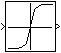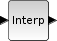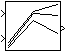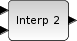# Xcos vs. Simulink® – Lookup tables library conversion

In the table below you can find the equivalent between the Simulink® and Xcos blocks for Lookup tables library/palette. The block which are not present in both libraries are omitted from the table.

This conversion table can be used in case you need to convert a Simulink® model to an Xcos block diagram model or vice-versa.

 Simulink Lookup tables library Xcos Lookup tables palette Xcos block descriptionLookup TableInterpolation The output of this block is a function of the input obtained by linear interpolation. This block has a single scalar input and a single scalar output port. The coord. and coord. give respectively the coordinate and the coordinate of the data points to be interpolated. The parameter vectors should be sorted (strictly increasing).Lookup Table (2-D)2D Interpolation The output of this block is a function of the inputs obtained by bilinear interpolation. This block has two scalar inputs and a single scalar output. The X(i) and Y(i) give respectively the X coordinate and the Y coordinate of the i-th data point to be interpolated and Z(Y(i),X(i)) its value.

Interpolation functions are heavily used in embedded control algorithms, hardware in the loop (HiL) systems and control function development. They are mainly used to translate sensor characteristics (electrical to physical) or as calibration (tunable) parameters function of one or two variables.

For the simple (1-D) interpolation block we are going to use as example, the characteristic of a temperature sensor. This is the dependency of the measured temperature function of the voltage output of the sensor. Let’s suppose that we have a temperature sensor with the following characteristic:

 Output voltage [V] 0.2 0.4 1 1.3 1.9 2.3 2.7 3.5 3.8 4.2 4.7 5 Measured temperature [°C] -20 -15 -5 -1 4 10 17 34 42 57 79 100

Usually, the characteristic of a sensor is supplied by the manufacturer of the sensor.

Next, we are going to load the values in the Scilab workspace and also plot the voltage-temperature characteristic of the sensor. Copy the Scilab instruction below in a script (*.sce) file.

// Lookup table data and plot
voltage_X = [0.2 0.4 1 1.3 1.9 2.3 2.7 3.5 3.8 4.2 4.7 5];
temperature_Z = [-20 -15 -5 -1 4 10 17 34 42 57 79 100];
figure()
plot(voltage_X, temperature_Z,'LineWidth',3)
xgrid()
xlabel('$\text{Voltage} \quad [V]$','fontsize',3)
ylabel('$\text{Temperature} \quad [^\circ C]$','fontsize',3)
plot(4.25,59.2,'rs','MarkerSize',8,'MarkBackground','r')

Running the above Scilab instructions will output the following graphical window:

The red point represents the value of the temperature for an input voltage of 4.25 V. Looking at the voltage-temperature curve, the corresponding temperature should be around 60 °C. We will find the exact value by loading the voltage values (axis, breakpoints) and temperature values (map, output) into a 1-D interpolation block.

For the bi-dimensional Lookup table (2-D), we are going to use as example the fuel map of an internal combustion engine (ICE). This map is not coming from a real engine, it’s purpose is only to demonstrate how the (2-D) interpolation block works.

 Engine Torque [Nm] 100 200 300 400 500 Engine Speed [rpm] 500 18 17 19 20 24 1500 17 15 20 22 25 2500 18 16 21 23 27 3500 19 21 23 25 30

We’ll follow the same approach as for the sensor characteristic. With the Scilab instructions below we’re going to load the axes and map values in the Scilab workspace and plot the fuel map as a surface with the surf() function.

// Lookup table (2-D) data and plot
engineSpeed_X = [500 1500 2500 3500];
engineTorque_Y = [100 200 300 400 500];
fuelMass_Z = [ 18 17 19 20 24;
17 15 20 22 25;
18 16 21 23 27;
19 21 23 25 30];
figure()
surf(engineTorque_Y, engineSpeed_X, fuelMass_Z)
xlabel('Engine Torque [Nm]','fontsize',2)
ylabel('Engine Speed [rpm]','fontsize',2)
zlabel('Fuel Mass [mg/stroke]','fontsize',2)
xgrid()

By running the Scilab instruction above we get the following graphical window:

Let’s look at a simple example in which we create a Simulink® block diagram model, simulate it and display the results. We’ll recreate the equivalent model in Xcos and check if the results are the same.

### Simulink® block diagram model

The same data for axes (breakpoints) and maps (outputs) were used in the Matlab workspace as in Scilab.

For the 1-D interpolation block, for 4.25 V input we get a temperature of 59.2 °C. This is very close to the expected value of 60 °C.

Also the 2-D interpolation block works correctly, because for an input speed of 2000 rpm, we expect that the output fuel mass to be in the middle interval of 15 ... 16. Looking in the fuel map table above we can deduce that the interpolation shows the proper value.

### Xcos block diagram model

We follow the same approach for the Xcos block diagram model. Using equivalent blocks (see table above), we recreate the same functionality of the Simulink model.

The main difference is that the 2-D interpolation block is expecting first the engine torque values (columns) and second the engine speed values (rows). This is reversed compared with the Simulink block, in which the first input is for the rows breakpoints (axis) and the second input is for the column breakpoints (axis).

As you can see, the displayed values of the Xcos block diagram model are identical with the Simulink results. This proves that we can use Xcos as an alternative to Simulink, when we want to model 1-D and 2-D lookup tables.

For any questions, observations and queries regarding this article, use the comment form below.

Don’t forget to Like, Share and Subscribe!

#### Ad Blocker Detected

Dear user, Our website provides free and high quality content by displaying ads to our visitors. Please support us by disabling your Ad blocker for our site. Thank you!